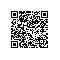# 【分享】Matlab R2015a 发布啦！

Matlab和C#混合编程文章目录：http://www.cnblogs.com/asxinyu/p/4329753.html

Matlab还是一如既往的每年2个版本的发布速度。2015年3月R2015a (版本 8.5) 正式发行。在新版本中，带有四个新的工具箱以及79个产品进行的更新。MATLAB 2015a 中的许多新功能都是在 R2014b 的功能基础上升级而来的，其中包括大数据增强功能、新的硬件支持，以及多种自定义工具箱的集成文档。请浏览下方功能，了解方功能，了解更多详细信息。

# 1.主要特性

• 用于数值计算、可视化和应用程序开发的高级语言
• 可实现迭代式探查、设计及问题求解的交互式环境
• 用于线性代数、统计、傅立叶分析、筛选、优化、数值积分以及常微分方程求解的数学函数
• 用于数据可视化的内置图形以及用于创建自定义绘图的工具
• 用于改进代码质量和可维护性并最大限度地发挥性能的开发工具
• 用于构建自定义图形界面应用程序的工具
• 可实现基于 MATLAB 的算法与外部应用程序和语言（如 C、Java、.NET 以及 Microsoft®Excel®）集成的函数

# 2.数值计算

MATLAB 提供了一系列用于分析数据、开发算法和创建模型的数值计算方法。MATLAB 语言包括用 以支持常见的工程设计和科学运算的数学函数。核心的数学函数采用处理器优化库，可以快速地执行向量运算和矩阵运算。

• 插值与回归
• 微分与积分
• 线性方程组
• 傅立叶分析
• 特征值与奇异值
• 常微分方程 (ODE)
• 稀疏矩阵

MATLAB 附加产品提供了各种专业领域的函数，如统计、优化、信号分析以及机器学习。

# 3.数据分析和可视化

MATLAB 提供了用于数据采集、分析和可视化的工具，使您能够深入探查数据，而且与使用电子表格或传统编程语言相比节省了大量时间。此外，还可以通过绘图、报告或发布MATLAB 代码的形式来记录和共享结果。

## 3.2 分析数据

利用 MATLAB，可以对数据进行管理、筛选以及预处理。您可以执行探索性数据分析，探明趋势，检验假设，并构建描述模型。MATLAB 提供了可用于滤波和平滑、插值、卷积以及快速傅 立叶变换 (FFT) 的各种函数。各种附加产品提供了可用于曲线和曲面拟合、多元统计、频谱分析、 图像分析、系统识别及其他分析任务的多种功能。

## 3.3 实现数据的可视化

MATLAB 提供了内置的二维和三维绘图函数，以及立体可视化函数。使用这些函数，可以实现数据可视化，了解数据，并交流结果。对绘图进行自定义时既可以采用交互方式，也可以采用编程方式。MATLAB 图例库提供了在 MATLAB 中以图形方式显示数据的各种示例。在每一个示例中，均可查 看和下载源代码，以便在 MATLAB 应用程序中使用。

## 3.4 存档和共享结果

您可以采用图形或完整报告的方式来共享结果。通过定制 MATLAB 图形，可以符合出版规格，并保存为常见的图形和数据文件格式。执行 MATLAB 程序时，可以自动生成报告。所生成的报告中含有代码、注释和程序结果，其中包括图形。报告可采用各种格式（如 HTML、PDF、Word 或 LaTeX）发布。

# 4.编程与算法开发

MATLAB 提供了一种高级语言和开发工具，使您可以迅速地开发并分析算法和应用程序。

## 4.1 MATLAB 语言

MATLAB 语言对向量运算和矩阵运算提供内在支持，这些运算是解决工程和科学问题的基础，能够实现快速开发和执行。使用 MATLAB 语言，编程和开发算法的速度较使用传统语言大幅提高，这是因为无须执行诸如声明变量、指定数据类型以及分配内存等低级管理任务。在很多情况下，支持向量运算和矩阵运算就无需使用 for 循环。因此，一行 MATLAB 代码通常等同于数行 C 代码或 C++ 代码。

MATLAB 提供了传统编程语言的多项功能，其中包括流控制、错误处理以及面向对象编程 (OOP)。您既可以使用基本的数据类型或高级数据结构，也可以定义自定义数据类型。采用交互方式，一次仅执行一个命令，可以即时生成结果。这种方法可以快速试探多个选项，通过反复迭代，找出最佳的解决方案。通过捕获交互式步骤，生成可以重复使用的脚本和函数，并实现任务的自动化。MATLAB 附加产品可针对信号处理和通信、图像和视频处理、控制系统以及许多其他领域提供各种内置算法。通过将这些算法与自己的算法结合使用，可以构建复杂的程序和应用程序。

## 4.2 开发工具

MATLAB 内置的各种工具可以实现高效的算法开发，包括：

• 命令行窗口 - 能够以交互的方式输入数据，执行命令和程序，以及显示结果
• MATLAB 编辑器 - 提供编辑和调试功能，如设置断点及逐步调试各行代码
• 代码分析器 - 自动检查代码是否有问题，并提出修改建议，以最大限度地发挥性能以及可维护性
• MATLAB 事件探查器 – 衡量 MATLAB 程序的性能，并确定需要修改加以改进的代码范围

## 4.3 与其他语言和应用程序集成

MATLAB 应用程序可以与其他语言编写的应用程序集成。在 MATLAB 中，可以直接调用以 C、C++、Java 和 .NET 编写的代码。使用 MATLAB 引擎库，可从 C、C++ 或 Fortran 应用程序调用 MATLAB 代码。

## 4.4 性能

MATLAB 采用处理器优化库，可以快速执行矩阵运算和向量运算。对于通用的标量计算，MATLAB 使用其即时 (JIT) 编译技术，提供了可与传统编程语言相媲美的执行速度。为了充分利用多核和多处理器计算机，MATLAB 提供了众多的多线程线性代数和数值函数。这些函数可在单个 MATLAB 会话中自动执行多个计算线程，从而得以在多核计算机上提高执行速度。通过附加的并行计算产品，可以充分利用多核台式机和其他的高性能计算资源，如 GPU 和群集。这些产品可提供高级构造，只需稍加改动 MATLAB 代码即可实现应用程序的并行化。

# 5.应用程序的开发和部署

MATLAB 工具和附加产品提供了一系列开发和部署应用程序的选项。您既可以与其他 MATLAB 用户共享各个算法和应用程序，也可以向其他没有 MATLAB 的用户实施免特许费的部署。

## 5.1 设计图形用户界面

使用 GUIDE（图形用户界面开发环境），可以布置、设计和编辑自定义图形用户界面。既可以 含带常用控件，如列表框、下拉菜单和按钮，也可以含带 MATLAB 绘图。此外，还可以使用 MATLAB 函数以编程的方式来创建图形用户界面。

## 5.2 部署应用程序

若要将一个应用程序直接分发给其他 MATLAB 用户，可以将其打包为 MATLAB 应用程序，从而 实现单文件分发。应用程序会自动安装在 MATLAB 应用程序库中，以便于访问。若要与没有 MATLAB 的其他人分享应用程序，可以使用应用程序部署产品。这些附加产品会自动 生成独立应用程序、共享库和软件组件，以便与 C、C++、Java、.NET 和 Excel 环境集成。可执行 文件和组件可以免特许费分发。MATLAB Production Server™ 使您能够在您的生产系统内运行由 MATLAB Compiler™ 打包的 MATLAB 程序，以便将数值分析融合到 Web、数据库和企业应用程序中。

## 5.3 生成 C 代码

使用 MATLAB Coder™，可以从 MATLAB 代码生成独立的 C 代码。MATLAB Coder 支持 MATLAB 语言的子集；设计工程师通常使用该语言来开发作为大型系统组件的算法。该代码既可用于独立执行，与其他软件应用程序集成，也可以作为嵌入式应用程序的一部分。

# 6.资源

MATLAB® 是受全球数百万工程师和科学家青睐的高级语言和交互环境。您可以探寻思路，实现可视化，开展跨学科协作，包括信号和图像处理、通讯、控制系统和计算金融。

1.正规的学术和商业途径。目前中国部分高校已经集中采购了Matlab的版权，可以去自己的学校查一下，有的话，可以通过正规渠道获取；

2.Matlab官方好像也提供学生版，自己注册下载看看，可能比较麻烦；

3.就是所谓的非正规途径了，自己使用搜索引擎获取看看，狂搜索，总归会有效的，有破解版；

4.至于其他方式，有需要的，应该懂的，3月30日中午12点前有效。使用钉钉扫一扫加入圈子
+ 订阅